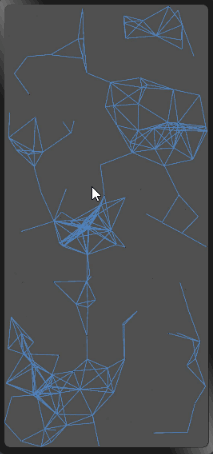# OpenHarmony - 基于ArkUI(JS)实现移动粒子效果背景## 效果演示## 实现步骤

### 1、创建canvas标签

`<div class="container">    <canvas ref="canvas1" style="width: 100%; height: 100%; background-color: rgb(80, 80, 80);" @touchmove="handleMove"></canvas></div>`

### 2、创建粒子

`export default {    data: {        dots: [], // 存储粒子对象的数组    },    // 创建粒子，并存储到数组中    drawBackground() {        // 创建粒子，并存储到数组中        for (let i=0; i<180; i++) {            // 粒子的位置            let x = Math.random() * 360            let y = Math.random() * 780            // 水平移动的速度，垂直移动的速度            let xa = Math.random() * 0.5            let ya = Math.random() * 0.5            this.dots.push({ x, y, xa, ya, max: 3600 })        }    },}`

### 3、手指触摸事件

web端的效果是跟随鼠标的移动，移动端没有鼠标，那我们就让粒子向手指移动的地方靠近。用手指触摸事件来模拟鼠标移动事件。

x代表手指触摸屏幕的横坐标，y代表手指触摸屏幕的纵坐标，max代表粒子向手指触摸屏幕位置靠近的最小距离条件。

handleMove事件的作用，手指触摸屏幕时更新手指的坐标位置。

`export default {    data: {        mouse: { // 手指位置            x: null,            y: null,            max: 3200        }    },    handleMove(e) {        this.mouse.x = e.touches.localX        this.mouse.y = e.touches.localY    }}`

### 4、粒子的运动

`draw(ctx) {    const self = this;    // 清空整个画布    ctx.clearRect(0, 0, 360, 780);    // 粒子和鼠标的结合，把鼠标数组添加到粒子数组中    const nDots = [this.mouse, ...this.dots];    // 粒子运动    this.dots.forEach(function (dot) {        dot.x += dot.xa;        dot.y += dot.ya;        // 粒子运动的边界（画布），反弹        dot.xa *= (dot.x > 360 || dot.x < 0) ? -1 : 1;        dot.ya *= (dot.y > 780 || dot.y < 0) ? -1 : 1;        // 绘制粒子        ctx.fillRect(dot.x, dot.y, 1, 1);        ctx.fillStyle = "#282828";        // 连线        for (let i=0; i<nDots.length; i++) {            let d = nDots[i];            if(d == dot) {                continue;            }            // 计算粒子的距离            let dx = dot.x - d.x;            let dy = dot.y - d.y;            let dDistance = Math.pow(dx, 2) + Math.pow(dy, 2);            // 连线操作            if (dDistance < d.max) {                // 处理触摸事件                if (d == self.mouse && dDistance > d.max / 2) {                    dot.x -= dx * 0.03;                    dot.y -= dy * 0.03;                }                // 绘制线条                ctx.beginPath();                ctx.lineWidth = 0.7;                ctx.strokeStyle = 'rgba(80, 130, 189, 0.9)';                // 起始位置                ctx.moveTo(dot.x, dot.y);                // 结束位置                ctx.lineTo(d.x, d.y);                ctx.stroke();                ctx.closePath();            }        }        // 删除比较过的粒子        nDots.splice(nDots.indexOf(dot), 1);    })},`

### 5、展示背景

`export default {    onShow() {        this.drawBackground();    },    drawBackground() {        // 创建粒子，并存储到数组中        for (let i=0; i<180; i++) {            // 粒子的位置            let x = Math.random() * 360;            let y = Math.random() * 780;            // 水平移动的速度，垂直移动的速度            let xa = Math.random() * 0.5;            let ya = Math.random() * 0.5;            this.dots.push({ x, y, xa, ya, max: 3600 }); // 两个粒子相吸的最小距离        }        const can = this.\$refs.canvas1;        const ctx = can.getContext('2d');        setInterval(() => {            this.draw(ctx);        }, 1000 / 60);    }}`

## 总结

1. 处理粒子向手指对象运动时，为了让速度不会太快，增加条件dDistance > d.max / 2。
2. 当粒子运动边界时，需要给粒子一个反方向的速度。
3. 在onInit生命周期中无法拿到canvas的dom，需要在onShow方法中获取。
4. 粒子运动的速度看起来不太柔和，需要调试下参数。51CTO技术栈公众号